# Planning: Prior distributions

## Bayesian inference

Bayesian statistics allows you to incorporate existing information into the sampling procedure and to revise this information using the information from the sample. The use of the prior distribution can potentially decrease the amount of audit work required to achieve the desired assurance, thereby increasing your efficiency as an auditor. For example, when you have information from the auditee’s internal controls that indicates a low-risk profile, you may build on this information to require less evidence from substantive testing. Be aware that all information that you incorporate into the statistical analysis should be justified.

### Prior probability distributions

Bayesian statistics incorporates existing information into the sampling procedure using a prior probability distribution. The prior distribution is a probability distribution that reflects your existing information about the misstatement in the population. Because the prior distribution is based on existing information, it is usually created before the auditor starts planning a sample.

## Constructing a prior distribution

What information can be incorporated into the prior distribution depends on what type of information is available, the quality of that information, and the situation at hand. When the auditor has decided what kind of information they want to incorporate into a prior distribution, they can use the auditPrior() function to calculate the corresponding parameters of the prior distribution. Below we discuss the various types of audit information that jfa is able to incorporate into a prior distribution.

First, we set some default options for the confidence, performance materiality, the likelihood, and the expected errors in the sample.

confidence  <- 0.95       # 95% confidence
likelihood  <- 'binomial' # Binomial likelihood
materiality <- 0.05       # Performance materiality of 5%
expected    <- 0.01       # 1% errors expected in sample

### Minimal information priors (method = 'default')

You can construct a prior distribution from minimal information using method = 'default'. As an example, the code below incorporates minimal information into a prior distribution.

prior1 <- auditPrior(method = 'default', likelihood = likelihood, expected = expected, conf.level = confidence)
summary(prior1)
##
##  Prior Distribution Summary
##
## Options:
##   Likelihood:                    binomial
##   Specifics:                     noninformative
##
## Results:
##   Functional form:               beta(a = 1, ß = 1)
##   Equivalent sample size:        1
##   Equivalent errors:             0
##   Mode:                          NaN
##   Mean:                          0.5
##   Median:                        0.5
##   Variance:                      0.083333
##   Skewness:                      0
##   95 percent upper bound:        0.95
##   Precision:                     NaN

You can visually inspect the prior distribution using the plot() function.

plot(prior1)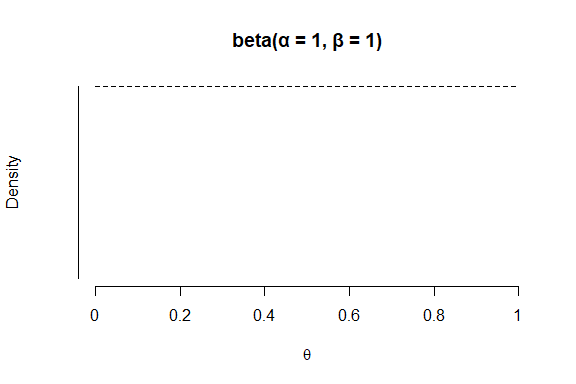### Prior distributions with classical properties (method = 'strict')

You can construct a prior distribution on the basis of no existing information using method = 'strict'. The prior distribution that is constructed is improper but yields exactly the same results as the classical methodology with respect to sample sizes, upper limits, and evidence.

prior2 <- auditPrior(method = 'strict', likelihood = likelihood)
summary(prior2)
##
##  Prior Distribution Summary
##
## Options:
##   Likelihood:                    binomial
##   Specifics:                     classical properties
##
## Results:
##   Functional form:               beta(a = 1, ß = 0)
##   Equivalent sample size:        0
##   Equivalent errors:             0
##   Mode:                          0
##   Mean:                          1
##   Median:                        1
##   Variance:                      0
##   Skewness:                      -Inf
##   95 percent upper bound:        1
##   Precision:                     1

You can visually inspect the prior distribution using the plot() function.

plot(prior2)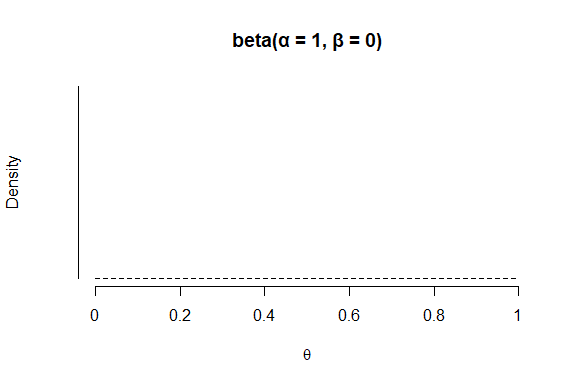### Custom prior distributions (method = 'param')

You can manually specify the $$\alpha$$ and $$\beta$$ parameters of the prior distribution using method = 'param' in combination with the alpha and beta arguments. As an example, the code below creates a beta(2, 10) prior distribution.

prior3 <- auditPrior(method = 'param', likelihood = likelihood, alpha = 2, beta = 10)
summary(prior3)
##
##  Prior Distribution Summary
##
## Options:
##   Likelihood:                    binomial
##   Specifics:                     a = 2; ß = 10
##
## Results:
##   Functional form:               beta(a = 2, ß = 10)
##   Equivalent sample size:        11
##   Equivalent errors:             1
##   Mode:                          0.1
##   Mean:                          0.16667
##   Median:                        0.14796
##   Variance:                      0.010684
##   Skewness:                      0.9214
##   95 percent upper bound:        0.36436
##   Precision:                     0.26436

You can visually inspect the prior distribution using the plot() function.

plot(prior3)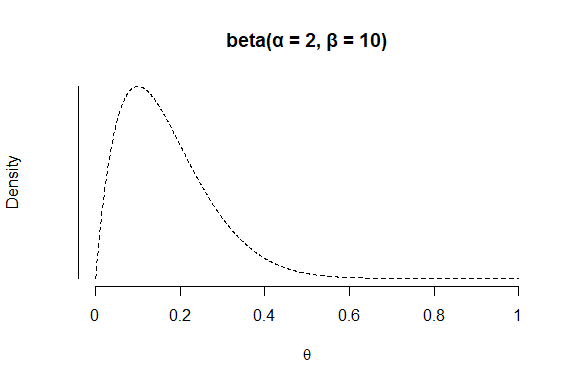### Prior distributions with equal prior probabilities (method = 'impartial')

You can incorporate the assumption that tolerable misstatement is equally likely as intolerable misstatement using method = 'impartial'. As an example, the code below incorporates this assumption into a prior distribution.

Note: This method requires that you specify a value for the materiality.

prior4 <- auditPrior(method = 'impartial', likelihood = likelihood, expected = expected, conf.level = confidence,
materiality = materiality)
summary(prior4)
##
##  Prior Distribution Summary
##
## Options:
##   Likelihood:                    binomial
##   Specifics:                     p(T < 0.05) = p(T > 0.05) = 0.5
##
## Results:
##   Functional form:               beta(a = 1.155, ß = 16.385)
##   Equivalent sample size:        16.54
##   Equivalent errors:             0.1554
##   Mode:                          0.01
##   Mean:                          0.065872
##   Median:                        0.049999
##   Variance:                      0.0033189
##   Skewness:                      1.5426
##   95 percent upper bound:        0.18118
##   Precision:                     0.17118

You can visually inspect the prior distribution using the plot() function.

plot(prior4)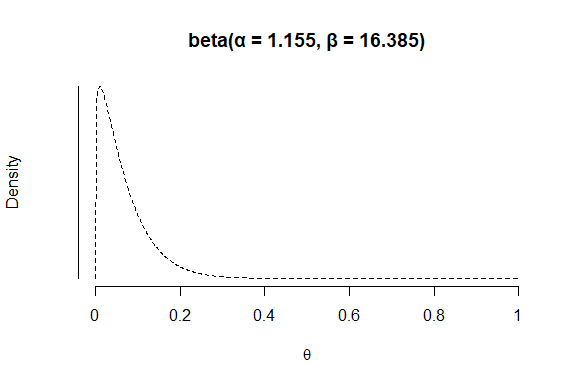### Prior distributions with custom prior probabilities (method = 'hyp')

You can manually assign prior probabilities to the hypothesis of tolerable misstatement and the hypotheses of intolerable misstatement (using p.hmin) in combination with method = 'hyp'. As an example, the code below incorporates the information that the hypothesis of tolerable misstatement has a probability of 60% into a prior distribution.

Note: This method requires that you specify a value for the materiality.

prior5 <- auditPrior(method = 'hyp', likelihood = likelihood, expected = expected, conf.level = confidence,
materiality = materiality, p.hmin = 0.6)
summary(prior5)
##
##  Prior Distribution Summary
##
## Options:
##   Likelihood:                    binomial
##   Specifics:                     p(T < 0.05) = 0.6; p(T > 0.05) = 0.4
##
## Results:
##   Functional form:               beta(a = 1.217, ß = 22.493)
##   Equivalent sample size:        22.71
##   Equivalent errors:             0.2171
##   Mode:                          0.01
##   Mean:                          0.051333
##   Median:                        0.039239
##   Variance:                      0.0019708
##   Skewness:                      1.5724
##   95 percent upper bound:        0.13983
##   Precision:                     0.12983

You can visually inspect the prior distribution using the plot() function.

plot(prior5)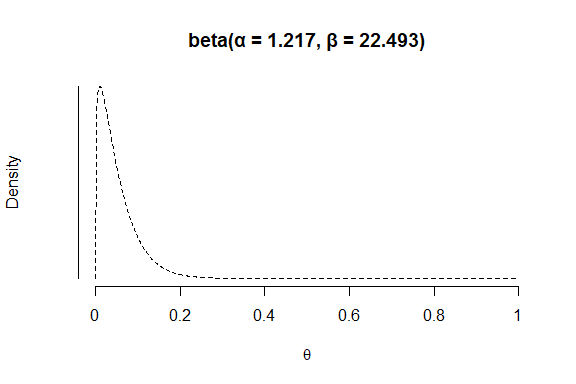### Prior distributions using the Audit Risk Model (method = 'arm')

You can translate the risk assessments from the Audit Risk Model (inherent risk and internal control risk) into a prior distribution using method = 'arm' in combination with the ir and cr arguments. As an example, the code below incorporates the information that the inherent risk is equal to 90% and that the internal control risk is equal to 60% into a prior distribution.

prior6 <- auditPrior(method = 'arm', likelihood = likelihood, expected = expected, conf.level = confidence,
materiality = materiality, ir = 0.9, cr = 0.6)
summary(prior6)
##
##  Prior Distribution Summary
##
## Options:
##   Likelihood:                    binomial
##   Specifics:                     ir = 0.9; cr = 0.6; dr = 0.0925926
##
## Results:
##   Functional form:               beta(a = 1.21, ß = 20.79)
##   Equivalent sample size:        21
##   Equivalent errors:             0.21
##   Mode:                          0.0105
##   Mean:                          0.055
##   Median:                        0.042056
##   Variance:                      0.0022598
##   Skewness:                      1.5602
##   95 percent upper bound:        0.14982
##   Precision:                     0.13932

You can visually inspect the prior distribution using the plot() function.

plot(prior6)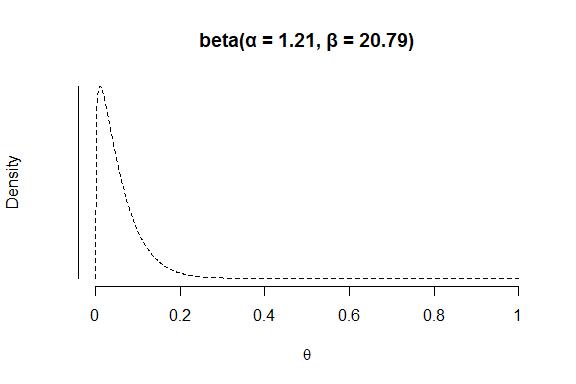### Prior distributions using the Bayesian Risk Assessment Model (method = 'bram')

You can incorporate information about the expected errors (mode) and the upper confidence bound of the prior distribution according to the Bayesian Risk Assessment Model (BRAM) using method = 'bram'. As an example, the code below incorporates the information that the mode of the prior distribution is 1% and the upper bound is 60%.

prior7 <- auditPrior(method = 'bram', likelihood = likelihood, expected = expected, conf.level = confidence,
materiality = materiality, ub = 0.6)
summary(prior7)
##
##  Prior Distribution Summary
##
## Options:
##   Likelihood:                    binomial
##   Specifics:                     mode = 0.01; upper bound = 0.6
##
## Results:
##   Functional form:               beta(a = 1.023, ß = 3.317)
##   Equivalent sample size:        3.34
##   Equivalent errors:             0.0234
##   Mode:                          0.01
##   Mean:                          0.23581
##   Median:                        0.19356
##   Variance:                      0.033746
##   Skewness:                      0.90737
##   95 percent upper bound:        0.5991
##   Precision:                     0.5891

You can visually inspect the prior distribution using the plot() function.

plot(prior7)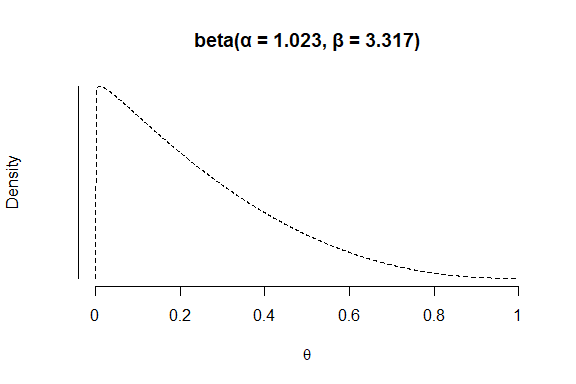### Prior distributions from an earlier sample (method = 'sample')

You can incorporate information from an earlier sample into the prior distribution using method = 'sample' in combination with x and n. As an example, the code below incorporates the information from an earlier sample of 30 items in which 0 misstatements were found into a prior distribution.

prior8 <- auditPrior(method = 'sample', likelihood = likelihood, expected = expected, conf.level = confidence,
materiality = materiality, x = 0, n = 30)
summary(prior8)
##
##  Prior Distribution Summary
##
## Options:
##   Likelihood:                    binomial
##   Specifics:                     earlier sample of 30 items with 0 errors
##
## Results:
##   Functional form:               beta(a = 1, ß = 30)
##   Equivalent sample size:        30
##   Equivalent errors:             0
##   Mode:                          0
##   Mean:                          0.032258
##   Median:                        0.02284
##   Variance:                      0.0009755
##   Skewness:                      1.8152
##   95 percent upper bound:        0.095034
##   Precision:                     0.095034

You can visually inspect the prior distribution using the plot() function.

plot(prior8)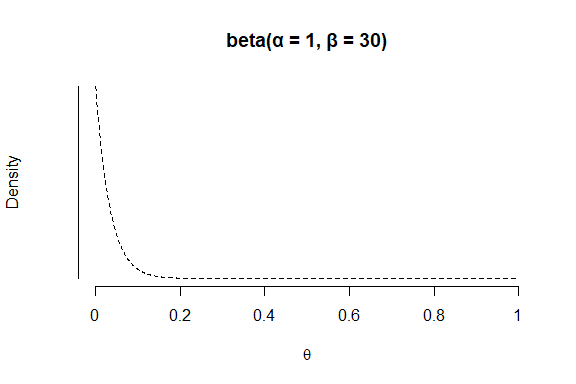### Prior distributions from a weighted earlier sample (method = 'factor')

You can incorporate information from last years results, weighted by a factor, into the prior distribution using method = 'factor' in combination with x and n. As an example, the code below incorporates the information from a last years results (a sample of 58 items in which 0 misstatements were found), weighted by a factor 0.7, into a prior distribution.

prior9 <- auditPrior(method = 'factor', likelihood = likelihood, expected = expected, conf.level = confidence,
materiality = materiality, x = 0, n = 58, factor = 0.7)
summary(prior9)
##
##  Prior Distribution Summary
##
## Options:
##   Likelihood:                    binomial
##   Specifics:                     earlier sample of 58 items with 0 errors weighted by 0.7
##
## Results:
##   Functional form:               beta(a = 1, ß = 40.6)
##   Equivalent sample size:        40.6
##   Equivalent errors:             0
##   Mode:                          0
##   Mean:                          0.024039
##   Median:                        0.016928
##   Variance:                      0.0005507
##   Skewness:                      1.8607
##   95 percent upper bound:        0.07113
##   Precision:                     0.07113

You can visually inspect the prior distribution using the plot() function.

plot(prior9)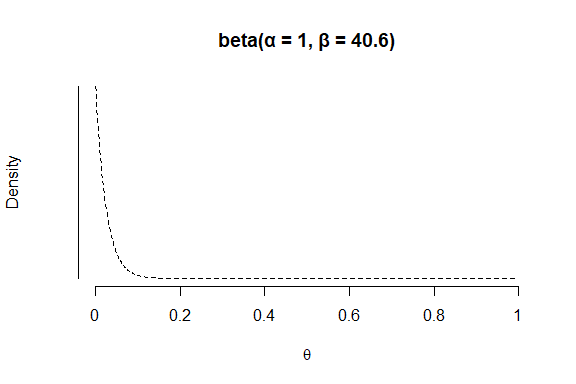## Using a prior distribution in other functions

Bayesian statistics allows the auditor to build on their existing information. Therefore, the objects returned by the auditPrior() function can be used as input for the prior argument in the planning() and evaluation() functions.

### Combining auditPrior() with planning()

The prior distribution can be used the planning stage to calculate a minimum sample size by providing the objected returned by the auditPrior() function to the planning() function. For example, the code below calculates the minimum sample size to test the misstatement in the population against a performance materiality of 5%, while incorporating the information in prior9.

jfa::planning(materiality = materiality, expected = expected, conf.level = confidence, prior = prior9)
##
##  Bayesian Audit Sample Planning
##
## minimum sample size = 29
## sample size obtained in 30 iteration(s) via method 'binomial' + 'prior'

### Combining auditPrior() with evaluation()

The prior distribution can be used the evaluation stage by providing the objected returned by the auditPrior() function to the evaluation() function. For example, the code below evaluates the misstatement in the population with respect to the performance materiality of 5% after seeing a sample of 60 items with 1 misstatement, while incorporating the information in prior8.

jfa::evaluation(materiality = materiality, conf.level = confidence, x = 1, n = 60, prior = prior9)
##
##  Bayesian Audit Sample Evaluation
##
## data:  1 and 60
## number of errors = 1, number of samples = 60, taint = 1, BF10 = 3.7968
## alternative hypothesis: true misstatement rate is less than 0.05
## 95 percent credible interval:
##  0.00000000 0.04628728
## estimate:
##  0.01004016
## estimates obtained via method 'binomial' + 'prior'